# Electronics and Communication Engineering - Microwave Communication

### Exercise :: Microwave Communication - Section 7

1.

In terms of R, L, G and C the propagation constant γ of a transmission line is

 A.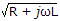B.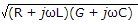C.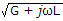D.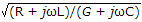Explanation:

No answer description available for this question. Let us discuss.

2.

The p mode of operation of magnetron signifies that

 A. the phase difference between adjacent anode poles is p radians B. the phase difference between adjacent anode poles is a multiple of p radians C. the total phase shift around the periphery is p radians D. none of the above

Explanation:

No answer description available for this question. Let us discuss.

3.

Consider the following statements (L is the length of array between centres of end antennas and A is wave-length)

1. Width of major lobe between nulls of broad side array and end fire array are different.
2. Directive gain of broadside array and end fire array are different.
3. Directive gain of broadside array is 4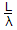Which of the above statements are correct?

 A. 1, 2 and 3 B. 1 and 2 only C. 1 only D. 1 and 3 only

Explanation:

No answer description available for this question. Let us discuss.

4.

The electrical requirement on wall thickness of rectangular wave guide is

 A. wall thickness is more than skin depth B. wall thickness is less than skin depth C. wall thickness is equal to skin depth D. either (b) or (c)

Explanation:

No answer description available for this question. Let us discuss.

5.

If a microstrip is enclosed in a metal enclosure

 A. the radiation losses are increased B. the radiation losses are reduced RL C. the capacitance becomes zero D. the inductance becomes zero